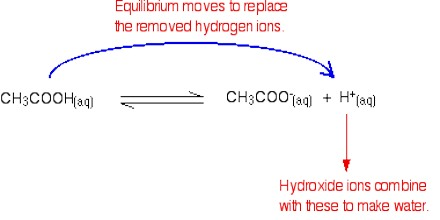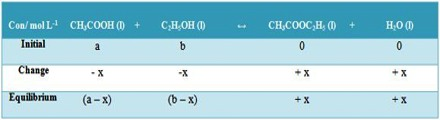Chemistry

# Equilibria in SolutionEquilibria in Solution

Applications of the equilibrium constants in solutions are numerous in connection with ionic equilibria. Such equilibria are also of great importance in dealing with biological phenomena. Here some non-ionic equilibria will be discussed. Of historical interest is the reaction between ethanoic acid and ethyl alcohol producing ethyl acetate and water according to the equation all components being in the liquid phase.

CH3COOH (l) + C2H5OH (l) ↔ CH3COOC2H5 (l) + H2O (l)Mixtures of known quantities of the acid and alcohol or ester and water are placed in sealed tubes and kept in a constant temperature bath for sufficiently long time to ensure attainment of equilibrium. This can be tested by occasional withdrawal of a flied volume of the mixture and analysis for acid content. This is generally done by titrating with standard NaOH solution after diluting the reaction mixture to a large volume to stop the reaction. When equilibrium has been established no change in the acid concentration can be detected. A complete analysis is then made and the quantities of acid and alcohol reacted are evaluated. The equilibrium constant, KC, is then given by;

KC = [CH3COOC2H5] [H2O] / [CH3COOH] [C2H5OH]

KC can calculated from the following concentration data in mol L-1.Then, KC = [(x) (x)] / [(a – x)(b – x)] = x2 / [(a – x)(b – x)]